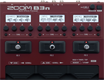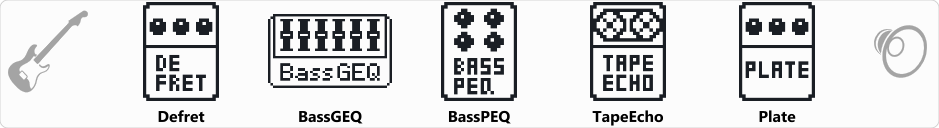# AcBassB3

Discussion in 'Zoom B3n' started by hooham482, Apr 27, 2021.

1. AcBassB3Device: Zoom B3n
Firmware: 2.00

Name on device: AcBassB3
Optimized for: Guitar Amp

Effects chain:B3 patch for acoustic bass sound. www.talkbass.comthreadslooking-for-zoom-b3-patch-for-acoustic-bass-sound.1220074

Effect: "Defret" (Sfx), active - "yes"
"Sense" = 23
"Color" = 1
"Tone" = 10
"Volume" = 80

Effect: "BassGEQ" (Filter), active - "yes"
"50Hz" = 0.0
"120Hz" = 3.0
"400Hz" = 2.5
"500Hz" = 0.0
"800Hz" = 3.0
"4.5kHz" = 0.0
"10kHz" = 0.0
"Volume" = 80
"50Hz" = 0.0
"120Hz" = 0.0
"400Hz" = 0.0
"500Hz" = 0.0
"800Hz" = 0.0
"4.5kHz" = 0.0
"10kHz" = 0.0
"Volume" = 80
"Parameter set" = A

Effect: "BassPEQ" (Filter), active - "yes"
"Frequency" = 250Hz
"Q" = 4.0
"Gain" = 0.0
"Volume" = 80

Effect: "TapeEcho" (Delay), active - "yes"
"Time" = 48
"F.B." = 5
"Mix" = 0
"Tail" = On

Effect: "Plate" (Reverb), active - "yes"
"PreD" = 200
"Decay" = 64
"Mix" = 56
"Tail" = Off

Patch Volume: 100